## Average Power Formula:

To find the average value of any power function, we have to take a particular time interval from t1 to t2; by integrating the function from t1 to t2 and dividing the result by the time interval t2 — t1, we get the Average Power Formula.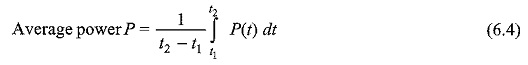In general, the average value over one cycle is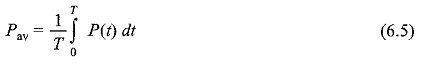By integrating the instantaneous power P(t) in Eq. 6.5 over one cycle, we get average power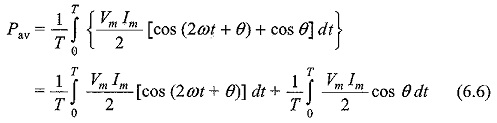In Eq. 6.6, the first term becomes zero, and the second term remains. The average power is therefore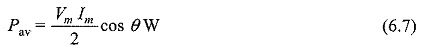We can write Eq. 6.7 as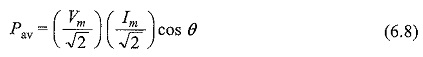In Eq.6.8, Vm/√2 and Im/√2 are the effective values of both voltage and current.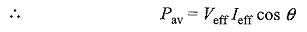To get average power, we have to take the product of the effective values of both voltage and current multiplied by cosine of the phase angle between voltage and the current.

If we consider a purely resistive circuit, the phase angle between voltage and current is zero. Hence, the average power is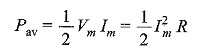If we consider a purely reactive circuit (i.e. purely capacitive or purely inductive), the phase angle between voltage and current is 90°. Hence, the average power is zero or Pav = 0.

If the circuit contains complex impedance, the average power is the power dissipated in the resistive part only.

Scroll to Top